Select Page

All above ground and below ground horizontal drainage pipes should be laid to

Gradients from 1 in 40 to 1 in 110 will normally give adequate
flow velocities.

A gradient of 1 in 80 is suitable for commencing calculations for pipe
schemes.

If a gradient is too steep i.e. steeper than 1 in 40, the liquid may
run faster than the solids in the sloping foul water pipe thus leaving the
solids stranded, which could then block the pipe.

If the gradient is not steep enough, i.e. less than 1 in 110, then the
pipe could still block if the solids slow down and become stranded.The fall in a
pipe may be defined as the vertical amount by which the pipe drops over a
distance. The distance can be between sections of pipe or between manholes. The
diagram below show pipe fall and distance.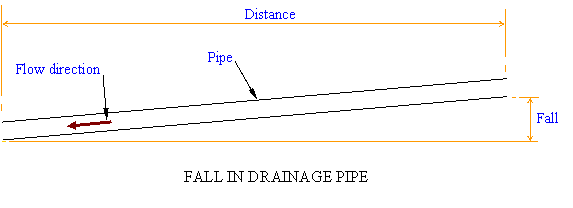A gradient may be defined as fall divided by distance.

For example is a 24 metre section of drainage pipe has a fall of 0.30 metres,

This can be converted into a gradient written as a ratio or 1: some
number.

Gradient = 1 / 0.0125 = 80

The above formula may be rearranged for Fall if the gradient is known:

### FALL = GRADIENT X DISTANCE

For example, calculate the fall in a 50 metre section of foul water pipework
if the gradient is to be 1 in 80.

A gradient of 1 in 80 is converted to a number instead of a ratio.

1 / 80 = 0.0125

### Fall = 0.0125 x 50

Fall = 0.625 metres or 625mm.### Invert Levels

The Invert Level of a pipe is the level taken from the bottom of the
inside of the pipe as shown below.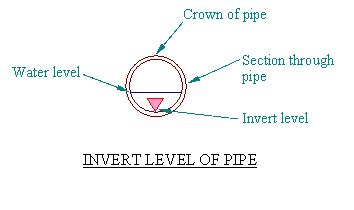The level at the crown of the pipe is the Invert level plus the
internal diameter of the pipe plus the pipe wall thickness. It may be necessary
to use this in calculations when level measurements are taken from the crown of
a pipe.

### Manholes

A manhole or access chamber is required to gain access to a drainage
system for un-blocking, cleaning, rodding or inspection. A typical manhole is
shown below.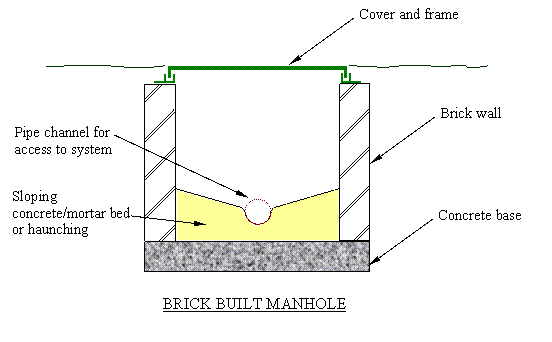Manholes may be manufactured from masonry or precast concrete. Sometimes
several precast concrete rings are used to form a manhole which speeds up the
on-site construction process. Normally deep manholes below 1.0 metre in
depth
require step irons to assist access for a workman.

Manholes and access chambers are also manufactured in PVC. An access chamber
is not usually large enough to admit a person but is suitable for access by
cleaning rods or hose and they are used for domestic applications, a common size
of plastic access chamber is 450mm diameter. For the domestic market plastic,
fibreglass or galvanised steel lids may be used but cast iron lids are required
where traffic crosses.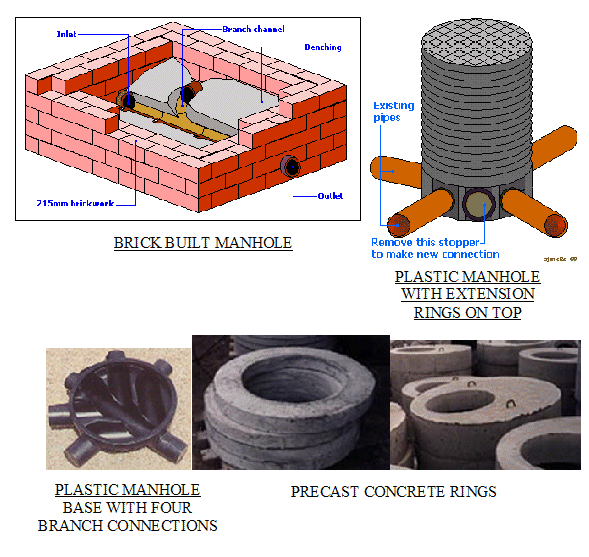A back drop manhole is used in areas where the surface level slopes as shown
below.

If the undergroung sewer pipe is to stay below ground it must follow the
average gradient of the slope. This invariably means that the pipe gradient
becomes too steep
, resulting in the solids being left stranded in the
pipe therefore causing a blockage.

To overcome this problem the back drop manhole was developed, as shown
below.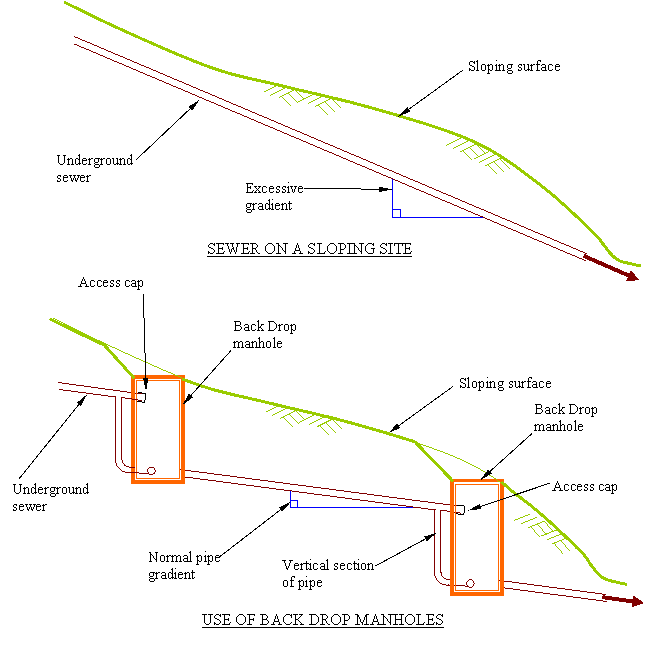An easier way to construct a back drop manhole is to use an internal vertical
section of pipe as shown below.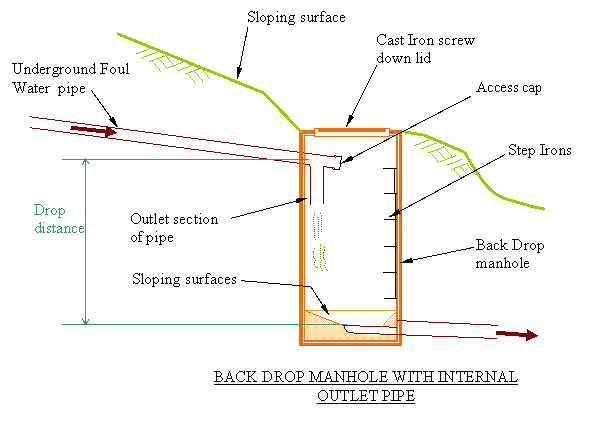Additional information and guidance is available on the Planning Portal website at